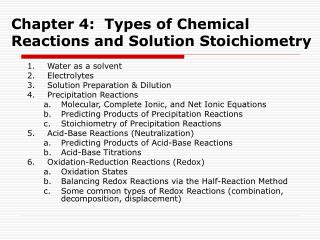DownloadDownload PresentationChapter 4: Types of Chemical Reactions and Solution Stoichiometry

# Chapter 4: Types of Chemical Reactions and Solution Stoichiometry

Download Presentation## Chapter 4: Types of Chemical Reactions and Solution Stoichiometry

- - - - - - - - - - - - - - - - - - - - - - - - - - - E N D - - - - - - - - - - - - - - - - - - - - - - - - - - -
##### Presentation Transcript

1. Chapter 4: Types of Chemical Reactions and Solution Stoichiometry • Water as a solvent • Electrolytes • Solution Preparation & Dilution • Precipitation Reactions • Molecular, Complete Ionic, and Net Ionic Equations • Predicting Products of Precipitation Reactions • Stoichiometry of Precipitation Reactions • Acid-Base Reactions (Neutralization) • Predicting Products of Acid-Base Reactions • Acid-Base Titrations • Oxidation-Reduction Reactions (Redox) • Oxidation States • Balancing Redox Reactions via the Half-Reaction Method • Some common types of Redox Reactions (combination, decomposition, displacement)

2. Example 1 Explain how you would prepare 500.0 mL of 0.250 M AgNO3 from solid AgNO3 and water. Answer: Weigh out 21.2 g of AgNO3, place it in a 500-mL volumetric flask, fill about half way and swirl to dissolve, then fill with water to calibration line.

3. Example 2 Explain how you would prepare 2.00 L of 0.100M HCl from 12.0M HCl. Answer: Measure out 16.7 mL of the 12.0M HCl and place it in a 2.00 L volumetric flask, then add water to the calibration line on the flask.

4. Example 3 How many grams of table salt are present in 100.0 mL of a 0.500M solution? Answer: 2.92 g NaCl

5. Example 4 Rust stains can be removed by washing a surface with a dilute solution of oxalic acid, H2C2O4.  The reaction is: Fe2O3(s) + 6 H2C2O4(aq) 2 Fe(C2O4)33-(aq) + 3 H2O (l) +  6 H+ (aq) What mass of rust, Fe2O3, can be removed by 1.0 L of a 0.14 M solution of oxalic acid? Answer: 3.7 g of rust can be removed.

6. Solubility Rules

7. Example 5 Predict the products of the following reactions and write balanced molecular, complete ionic, and net ionic equations for each. Ba(NO3)2 (aq) + K2SO4 (aq) (NH4)3PO4(aq) + AlCl3(aq) NaI (aq) + Al2(SO4)3(aq)

8. Example 6 If 25.0 mL of 0.200 M AgNO3 is mixed with 15.0 mL of 0.500 M MgCl2, what mass of precipitate is formed? What is this precipitate? Answer: 0.717 g of AgCl is formed

9. Example 7 A 50.00 mL sample of an H2SO4 solution of unknown concentration was titrated with 1.993 M NaOH. A volume of 84.14 mL of the NaOH solution was needed to reach the endpoint. What is the molarity of the H2SO4 solution? Answer: 1.677 M H2SO4

10. Example 8 An antacid tablet contains CaCO3 and inert ingredients.  A student dissolves a 0.522g sample of this antacid in 50.0 mL of 0.1077M HCl.  After the reaction is complete, the excess acid remaining in the solution is titrated with 0.1109M NaOH.  If it takes 13.2 mL of the NaOH solution to reach the equivalence point, find the mass percent of CaCO3 in the antacid tablet. Answer: 37.6% CaCO3 in the antacid tablet

11. Rules for Assigning Oxidation Numbers Click here for the Rules for Assigning Oxidation Numbers handout.

12. Example 9 Assign oxidation states to each atom in the following compounds: CaCl2 K3PO4 C3H8 K2Cr2O7

13. The Half Reaction Method for Balancing Redox Reactions Click here for the Half Reaction Method handout

14. Example 10 Balance the following redox reactions: • H2S + HNO3 S8 + NO • Cr2O7 2- + I- Cr3+ + IO3- (acidic) • CrI3 + Cl2 CrO42- + IO4- + Cl- (basic)

15. Example 11 A sample of copper ore weighing 0.4000 g was dissolved in nitric acid to produce a solution of Cu2+ ions. An excess of potassium iodide was added to this solution, producing iodine and a precipitate of copper (I) iodide: 2 Cu2+(aq) + 4I-(aq) 2 CuI (s) + I2(aq) The iodine, I2, was then titrated with 0.1056M sodium thiosulfate solution (Na2S2O3). In the titration, I2 is reduced to I- and S2O32- is oxidized to S4O62-. The sodium is merely a spectator ion. If 24.65 mL of the thiosulfate solution are required to reach the endpoint, what is the percent by mass of copper in the ore? Answer: 41.35%